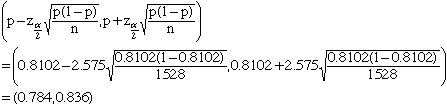Kim senior student Question: A poll asked 1528 adults if they were in favor of the death penalty, 1238 said yes, find 99% confidence level for percent of all adult who are in favor of the death penalty. Hi Kim, Since n=1528 is very large, for confidence intervals for proportions we can use Z-test. Estimation of proportion p=1238/1528 = 0.8102, aplha/2 = (1-.99)/2=.005, z(aplha/2)=2.575 (found by the table of areas under the standard normal probability distribution). So confidence interval isor in percent (78.4%, 83.6%) Andrei Go to Math Central